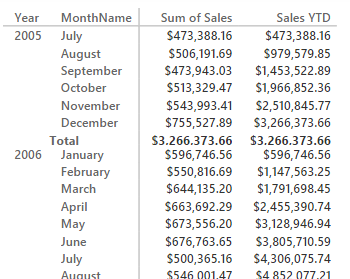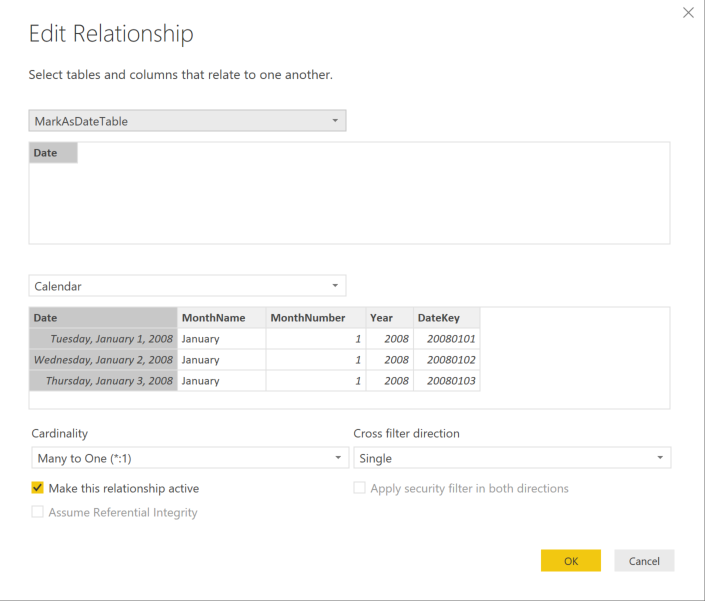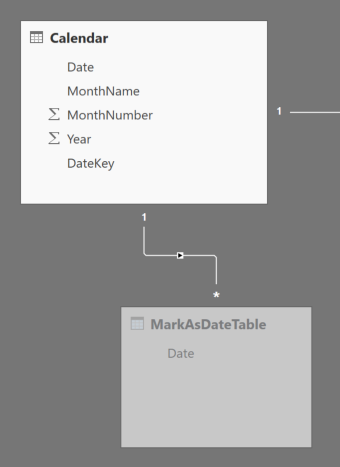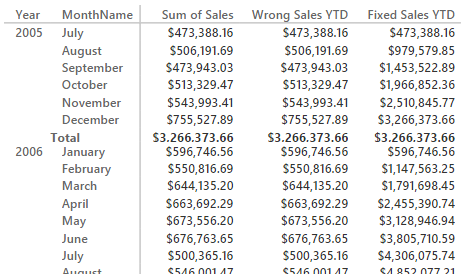UPDATE 2018-02-06 : the February 2018 release of Power BI Desktop introduced the Mark as Date Table feature. Thus, the content of this article is now obsolete because you can activate the feature that was missing in Power BI. We keep the content available as a reference. It is still relevant if you use older versions of Power BI Desktop.

UPDATE 2017-02-22 : there is a new technique described in the section “Adding a Dummy Fact table” that describes how to obtain the behavior of Mark As Date Table in Power BI with a minimal effort.

When you create a model in Power Pivot or Analysis Services Tabular, you can apply the setting “Mark as Date Table” choosing a column of Date data type as the date in the table. You can have many date tables in a single data model and this setting affects both the metadata read by the clients (which can provide a particular user interface to manipulate a date selection) and the behavior of certain DAX expressions that manipulates filters in a date table.

Because this setting is not present in new models created in Power BI Desktop (this article will be updated in the future as soon as this feature will be available), you might not apply to your data model all the existing time intelligence functions available in DAX. This issue is not present if create the Power BI Desktop model importing an existing Power Pivot data model with the Mark as Date Table setting active. However, several workarounds are possible, once you are aware of the behavior of this setting in DAX.

## Time Intelligence Functions in DAX

The DAX language provides a number of functions for Time Intelligence (https://support.office.com/en-us/article/Time-Intelligence-in-Power-Pivot-in-Excel-016ACF7B-9DED-411E-BA6C-ED8B8C368011). These functions can be divided in two categories:

• Functions that returns a scalar value without requiring CALCULATE
• Functions that returns a table, which has to be used as a filter in a CALCULATE statement

An example of the first group is TOTALYTD. This group, in reality, only simplifies the writing of a corresponding CALCULATE expression using a time intelligence function included in the second group. For example, if you write an expression using TOTALYTD:

```TOTALYTD (
SUM ( Sales[SalesAmount] ),
'Calendar'[Date]
)
```

In reality you are writing a CALCULATE statement which has a DATESYTD in the filter argument:

```CALCULATE (
SUM ( Sales[SalesAmount] ),
DATESYTD ( 'Calendar'[Date] )
)
```

This last expression applies a filter to the Calendar[Date] column, which replaces an existing filter in that column (and in other columns of the Calendar table most of the times, as we will see later). In practice the DATESYTD function can be replaced by a FILTER, and the previous expression corresponds to the following one:

```CALCULATE (
SUM ( Sales[SalesAmount] ),
FILTER (
ALL ( 'Calendar'[Date] ),
'Calendar'[Date] <= MAX ( 'Calendar'[Date] )
&& YEAR ( 'Calendar'[Date] ) = YEAR ( MAX ( 'Calendar'[Date] ) )
)
)
```

If you know how the filter context (https://www.sqlbi.com/articles/row-context-and-filter-context-in-dax/) works, you might wonder why the filter over a single date column removes the filter on other columns (such as Year and Month), as it happens when you use the measure for year-to-date using the expression above in a report.In fact, in order to remove all the filters from other columns, you might write the following expression, which only differ from the previous one because the filter iterates the entire Calendar table and not only the values of the Date column in the same table.

```CALCULATE (
SUM ( Sales[SalesAmount] ),
FILTER (
ALL ( Calendar ),
'Calendar'[Date] <= MAX ( 'Calendar'[Date] )
&& YEAR ( 'Calendar'[Date] ) = YEAR ( MAX ( 'Calendar'[Date] ) )
)
)
```

Here is the where the Mark as Data Table setting can make a difference.

## The Mark as Date Table Setting

When you apply the Mark as Date Table setting to a table, the DAX engine automatically adds an ALL function over the same table in each CALCULATE statement where a filter over the column used in that setting. For example, if you marked a table named Calendar as a date table using the Date column (yes, too many Date names – in practice, you have a column called Calendar[Date]), and you can write the following expression:

```CALCULATE (
SUM ( Sales[SalesAmount] ),
DATESYTD ( 'Calendar'[Date] )
)
```

The DAX engine automatically adds an ALL function over the Calendar table, removing any existing filter on other columns of the same table:

```CALCULATE (
SUM ( Sales[SalesAmount] ),
DATESYTD ( 'Calendar'[Date] ),
ALL ( 'Calendar' )
)
```

However, this ALL statement is automatically applied when you apply a filter over a column of Date type that is the primary key in a relationship, regardless of the presence of the Mark as Date Table setting in the Calendar table. For this reason, you might observe that time intelligence functions sometime work also when the Mark as Date Table setting is not active, because the Date column is used in the relationship with other tables.

If you have a Calendar table that is related to other tables using a column that is not of Date data type, you have to either use the Mark as Date Table setting or use append the ALL ( Calendar ) function call in the filter arguments of CALCULATE.

## Scenarios in Power BI Desktop

In Power BI Desktop you can use all the time intelligence functions available in DAX when the Calendar table has relationships with other tables using a column of Date data type. However, you might often have tables that do not have a date column, but use an integer or a string column instead. This is the typical case of a data mart with surrogate keys, that are often expressed using an integer containing the date in the format YYYYMMDD. In order to simplify the following description, we will call this column a “surrogate key”, regardless of the fact it comes from a data mart or not.

In this case, since you do not have the Mark as Date Table setting available in Power BI Desktop user interface, you have to rely on one of the followings possible workarounds. You will find examples of Power BI Desktop models in the zip file you can download.

## Adding a Date Column in the Related Table

If you have a date column in the Calendar table that is not used as a key in the relationship with other tables, you can create a Date column in the other tables and then create a relationship using this column instead of the non-Date column. You can do this operation in the original query (if the data source if a relational database), or in the query in Power BI Desktop (using the Merge function between the two tables).

You cannot use a calculated column because of the interference of hidden date tables created by Power BI Desktop automatically. This approach could be expensive if you have to join a large fact table with the Calendar table in the source query. However, using this solution, all the time intelligence functions available will work regularly.

## Adding a Dummy Fact table

Section added on 2017-02-22 : you can create a calculated table (named MarkAsDateTable) using the following formula:

```MarkAsDateTable =
FILTER (
DATATABLE( "Date", DATETIME, { { BLANK() } } ),
FALSE
)
```

Then, hide the MarkAsDateTable table and create a relationship between the Calendar table and the new MarkAsDateTable. The relationship must be configured as in the following screenshot:The final result is the relationship that you see in the following picture:At this point, the Date column in the Calendar table is considered a primary key and applying a filter on it automatically generates the ALL ( Calendar ) condition that is required for time intelligence functions to work. You can find this working example in the Power BI file “Time Intelligence with Surrogate Key – fixed using hidden dummy fact table” included in the ZIP file that you can download.

## Adding an ALL function in all the DAX Measures

You can fix all the measures and other DAX expressions using time intelligence functions by removing the filter from all the columns of the date table using the ALL function. You can see an example in the following expression that fixes the year-to-date calculation.

```Fixed Sales YTD :=
CALCULATE (
SUM ( Sales[SalesAmount] ),
DATESYTD ( 'Calendar'[Date] ),
ALL ( 'Calendar' )
)
```

In the following picture, the Wrong Sales YTD corresponds to the definition of Sales YTD you have seen previously in this article.ALL” width=”467″ height=”274″ class=”aligncenter size-full wp-image-28231″ />

However, with this approach you cannot use the time intelligence function of the first group, which returns a scalar value (such as TOTALYTD) instead of a table to be used in a filter argument of a CALCULATE statement (such as DATESYTD). Thus, if you have TOTALYTD (or similar functions) you have also to convert them in the explicit CALCULATE version (using DATESYTD or corresponding functions).
UPDATE (thanks to the comment of Matthew Brice): With the time intelligence functions of the first group, such as TOTALYTD, you have to add the ALL ( ‘Calendar’ ) filter in the third argument.

## Importing the Data Model from Power Pivot

If you create the data model originally in Power Pivot, and you set the Mark as Date Table setting there, once you import the data model in Power BI, you can use all the time intelligence functions (including TOTALYTD and other scalar functions) even if the relationship does not use a column of Date data type. However, since you cannot import a Power BI Desktop data model in Power Pivot, you cannot apply this technique to an existing data model in Power BI, unless you rebuild it from scratch in Power Pivot.